. .
.
Free Vibration of a Cantilever Beam (Continuous System)
.
.

4.3  Mathematical Analysis

### For a cantilever beam subjected to free vibration, and the system is considered as continuous system in which the beam mass is considered as distributed along with the stiffness of the shaft, the equation of motion can be written as (Meirovitch, 1967),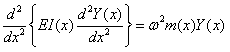(4.1)

### Where, E is the modulus of rigidity of beam material, I is the moment of inertia of the beam cross-section, Y(x) is displacement in y direction at distance x from fixed end, ω  is the circular natural frequency, m is the mass per unit length, m = ρA(x) ,  ρ is the material density,  x is the distance measured from the fixed end.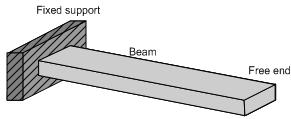Fig. 4.1 (a): A cantilever beam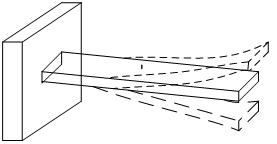Fig. 4.1 (b): The beam under free vibration

### We have following boundary conditions for a cantilever beam (Fig. 4.1)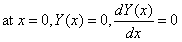(4.2)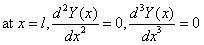(4.3)

### For a uniform beam under free vibration from equation (4.1), we get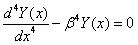(4.4)

### The mode shapes for a continuous cantilever beam is given as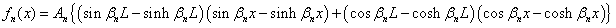(4.5)

### Where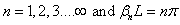### A closed form of the circular natural frequency ωnf, from above equation of motion and boundary conditions can be written as,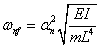(4.6)

### Where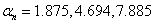### First natural frequency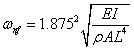(4.7)

### Second natural frequency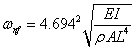(4.8)

### Third natural frequency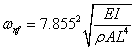(4.9)

### The natural frequency is related with the circular natural frequency as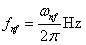(4.10)

### where I, the moment of inertia of the beam cross-section, for a circular cross-section it is given as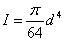(4.11)

### Where, d is the diameter of cross section and for a rectangular cross section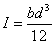(4.12)

### Where band d are the breadth and width of the beam cross-section as shown in the Fig. 4.2.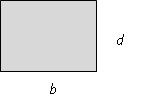Fig. 4.2: Cross-section of the cantilever beam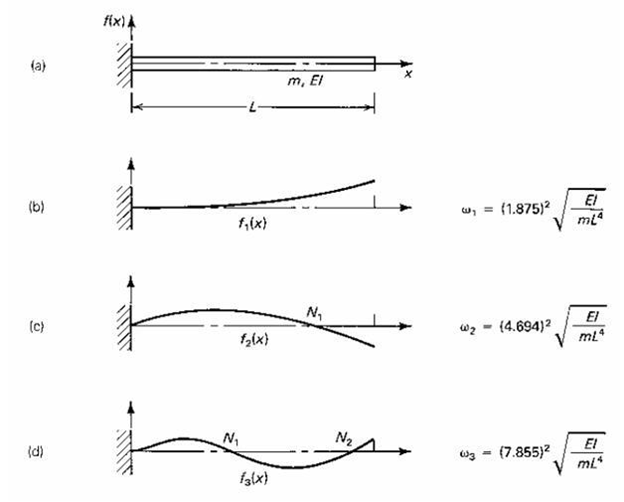Fig. 4.3: The first three undamped natural frequencies and mode shape of cantilever beam

Table 4.1  Material properties of various beams

 Material Density (kg/m3) Young’s Modulus (N/m2) Steel 7850 2.1×1011 Copper 8933 1.2×1011 Aluminum 2700 0.69×1011

Table 4.2  Different geometries of the beam

 Length,L,(m) Breadth,b,(m Depth,h,(m) 0.45 0.02 0.003 0.65 0.04 0.003

### First natural frequency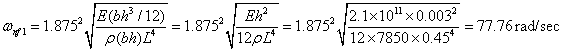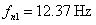### Second natural frequency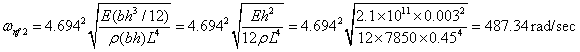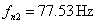### Third natural frequency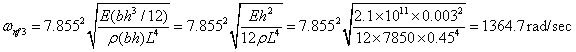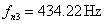### 1. First natural frequency - Let us consider the beam specimen as mass-less with stiffness k and has a discrete effective mass, meff , at the free end, which produces the same frequency as a continuous beam specimen without any tip mass. Hence, the natural frequency of discrete model of the beam without an accelerometer can be written as,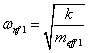(4.13)

### with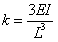(4.14)

### From which the effective mass at tip can be written as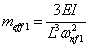(4.15)

### with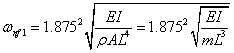### or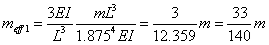### Now, if we consider the mass of accelerometer, macc, at the free end of the beam, than the total mass at free end will be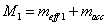(4.16)

### So for the discrete beam with accelerometer, the theoretical fundamental natural frequency after considering the mass of accelerometer will be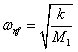(4.17)

### Taking data from Tables 4.1 and 4.2 for steel,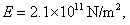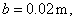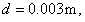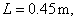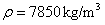,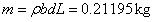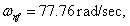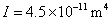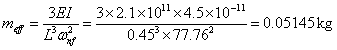### The mass of accelerometer is 4.8 gm = 0.0048 kg, so the total mass will be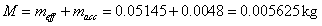### Hence the corrected natural frequency after consideration of mass of the accelerometer would be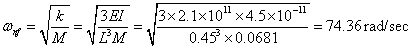### which can be written as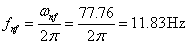### 5.      The last step is to find the solution from these modified equations.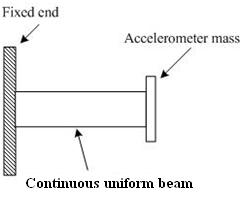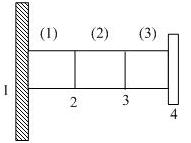Fig. 4.4 (a): A cantilever beam with a tip mass                Fig. 4.4 (b): Disceretisation of the beam

into 3 elements

### For a uniform beam, the elemental stiffness matrix (Tiwari, 2010; Dixit, 2009)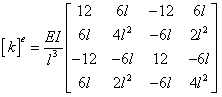(4.18)

### and the consistence mass matrix is given as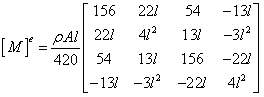(4.19)

### 2. Second step: Construct the elemental equations. For the first element, from equations (4.18) and (4.19), we have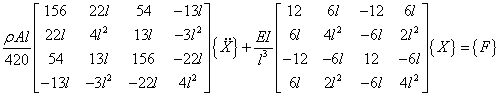(4.20)

### Then for the second element(4.21)

### In this only nodal variables will change and for third element, the elemental equations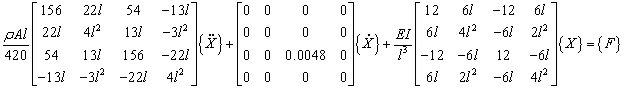(4.22)

### 3. Third step: Assemble all the elemental matrices to form a global matrix. The length of the each element  l = 0.45÷3 m and area is A = 0.002×0.03 m2, mass density of the beam material ρ = 7850 Kg/m3, and Young’s modulus of the beam E = 2.1 × 1011 N/m. After substituting values of the l, ρ, d, E, A in elemental equations (4.20), (4.21) and (4.22); assembled equations become,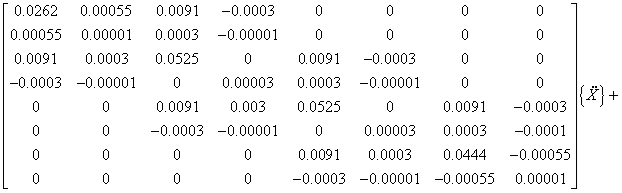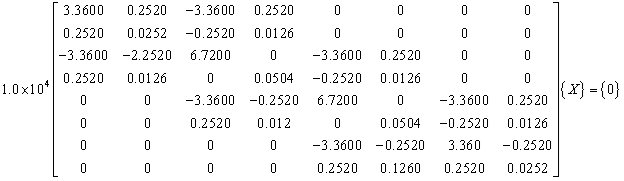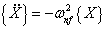### Hence, above equations can be written as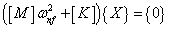(4.23)

### By solving the eigen value problem of equation (4.23), we get ωnf1 = 11.85 Hz and ωnf2 = 74.711 Hz. If we take more elements then we will get very accurate results especially of the higher modes. For example, on taking 10 elements then the first and second natural frequencies are Hz and Hz. From experimental response plot (Fig 4.5) of the same beam, the first natural frequency is 11Hz and second natural frequency is 79Hz. Some deviation is expected especially in higher mode due to modeling error of actual test conditions.

Cite this Simulator:

.....
..... .....Copyright @ 2023 Under the NME ICT initiative of MHRD